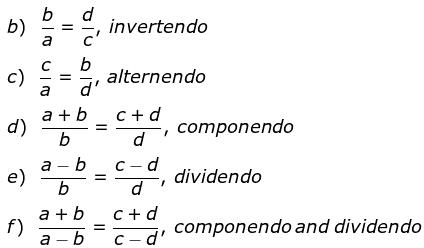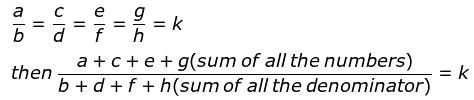Courses

# Formulae - Ratio & Proportion CAT Notes | EduRev

## CAT : Formulae - Ratio & Proportion CAT Notes | EduRev

The document Formulae - Ratio & Proportion CAT Notes | EduRev is a part of CAT category.
All you need of CAT at this link: CAT

Ratio, Proportions & Variations; Partnerships

Ratio and Proportions is one of the easiest concepts in CAT. It is just an extension of high school mathematics.

Questions from this concept are mostly asked in conjunction with other concepts like similar triangles, mixtures and alligations.

Hence fundamentals of this concept are important not just from a stand-alone perspective, but also to answer questions from other concept

1. Ratio is the comparison between similar types of quantities; it is an abstract quantity and does not have any units.

2. If a/b = c/d , then a, b, c, d are said to be in proportion.

3. If a/b = c/d, then
If a, b, x are positive, then
If a>b, then a+x/b+x <a/b
If a<b, then a+x/b+x >a/b
If a>b, then a−x/b−x >a/b
If a<b, then a−x/b-x<a/b

4.  If a/p= b/q = c/r =d/s =...,then a:b:c:d:...=p:q:r:s:...

(a) a × d = c × b5. If a, b, x are positive, then
If a>b, then a+x/b+x <a/b
If a<b, then a+x/b+x >a/b
If a>b, then a−x/b−x >a/b
If a<b, then a−x/b-x<a/b

6. If a/p= b/q = c/r =d/s =...,then a:b:c:d:...=p:q:r:s:...

7. If8. Types of ratios:

• Duplicate Ratio of a:b is a2 :b2
• Sub-duplicate ratio of a:b is √a: b
• Triplicate Ratio of a:b is a3 :b3
• Sub-triplicate ratio of a : b is a1/3 : b1/3

Product of proportions:

1. If a:b = c:d is a proportion, then

• Product of extremes = product of means i.e., ad = bc
• Denominator addition/subtraction: a:a+b = c:c+d and a:a-b = c:c-d
• a, b, c, d,.... are in continued proportion means, a:b = b:c = c:d = ....
• a:b = b:c then b is called mean proportional and b2 = ac
• The third proportional of two numbers, a and b, is c, such that, a:b = b:c ▪ d is fourth proportional to numbers a, b, c if a:b = c:d

Variations:

1. If a ∝ b, provided c is constant and a ∝ c, provided b is constant, then a ∝ b ∝ c,  if all three of them are varying.

2. If A and B are in a business for the same time, then Profit distribution ∝ investment (Time is constant).

3. If A and B are in a business with the same investment, then Profit distribution ∝ Time of investment (Investment is constant).

4. Profit Distribution  ∝ investment × Time.

Offer running on EduRev: Apply code STAYHOME200 to get INR 200 off on our premium plan EduRev Infinity!

,

,

,

,

,

,

,

,

,

,

,

,

,

,

,

,

,

,

,

,

,

;